# 【NLP】Attention机制与实战（Tensoflow2.x）

Attention是一种用于提升基于RNN（LSTM或GRU）的Encoder + Decoder模型效果的机制（Mechanism），一般称为Attention Mechanism。Attention给模型赋予了区分辨别的能力，例如，在机器翻译、语音识别应用中，为句子中的每个词赋予不同的权重，使神经网络模型的学习变得更加灵活（soft），同时Attention本身可以做为一种对齐关系，解释翻译输入/输出句子之间的对齐关系，解释模型到底学到了什么知识。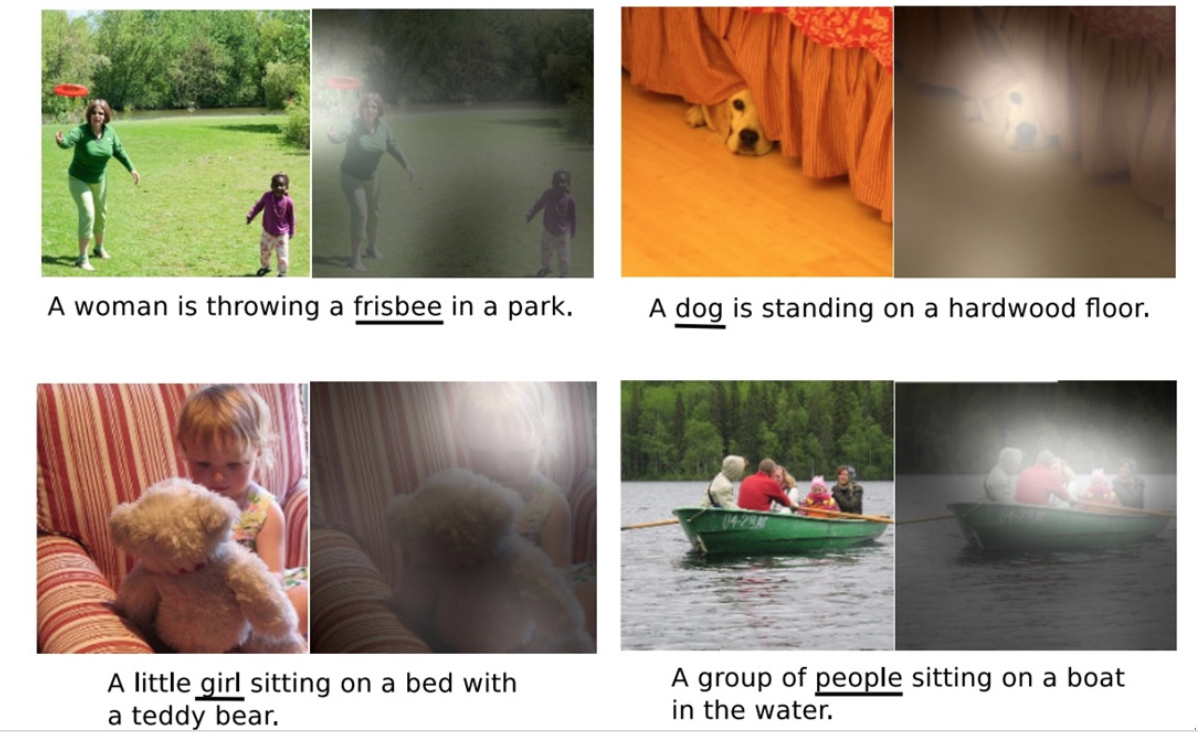Attention Mechanism与人类对外界事物的观察机制很类似，当人类观察外界事物的时候，一般不会把事物当成一个整体去看，往往倾向于根据需要选择性的去获取被观察事物的某些重要部分，比如我们看到一个人时，往往先Attention到这个人的脸，然后再把不同区域的信息组合起来，形成一个对被观察事物的整体印象。因此，Attention Mechanism可以帮助模型对输入的X每个部分赋予不同的权重，抽取出更加关键及重要的信息，使模型做出更加准确的判断，同时不会对模型的计算和存储带来更大的开销，这也是Attention Mechanism应用如此广泛的原因。

# 一、Attention Mechanism原理

## 1.1 Attention Mechanism主要需要解决的问题

《Sequence to Sequence Learning with Neural Networks》介绍了一种基于RNN的Seq2Seq模型，基于一个Encoder和一个Decoder来构建基于神经网络的End-to-End的机器翻译模型，其中，Encoder把输入X编码成一个固定长度的隐向量C，Decoder基于隐向量C解码出目标输出Y。这是一个非常经典的序列到序列的模型，但是却存在两个明显的问题：
1、把输入X的所有信息有压缩到一个固定长度的隐向量C，忽略了输入X的长度，当输入句子长度很长，特别是比训练集中最初的句子长度还长时，模型的性能急剧下降。
2、把输入X编码成一个固定的长度，对于句子中每个词都赋予相同的权重，这样做是不合理的，比如，在机器翻译里，对输入的每个词赋予相同权重，这样做没有区分度，往往使模型性能下降

## 1.2 Attention Mechanism原理

2014年在论文《Sequence to Sequence Learning with Neural Networks》中使用LSTM来搭建Seq2Seq模型。随后，2015年，Kyunghyun Cho等人在论文《Learning Phrase Representations using RNN Encoder–Decoder for Statistical Machine Translation》提出了基于GRU的Seq2Seq模型。两篇文章所提出的Seq2Seq模型，想要解决的主要问题是，如何把机器翻译中，变长的输入X映射到一个变长输出Y的问题，主要结构如下：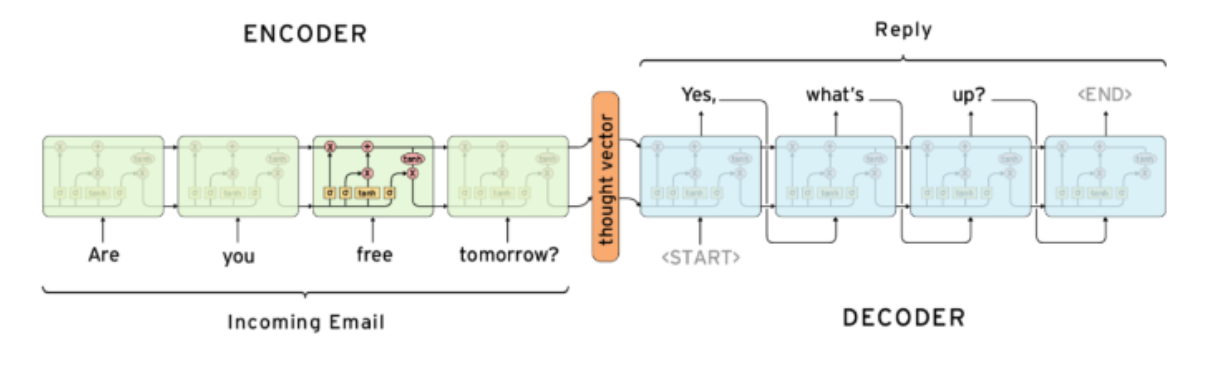Encoder把一个变成的输入序列x1，x2，x3…xt编码成一个固定长度隐向量（背景向量，或上下文向量context）c，c有两个作用：
1、做为初始向量初始化Decoder的模型，做为decoder模型预测y1的初始向量。
2、做为背景向量，指导y序列中每一个step的y的产出。Decoder主要基于背景向量c和上一步的输出yt-1解码得到该时刻t的输出yt，直到碰到结束标志（<EOS>）为止。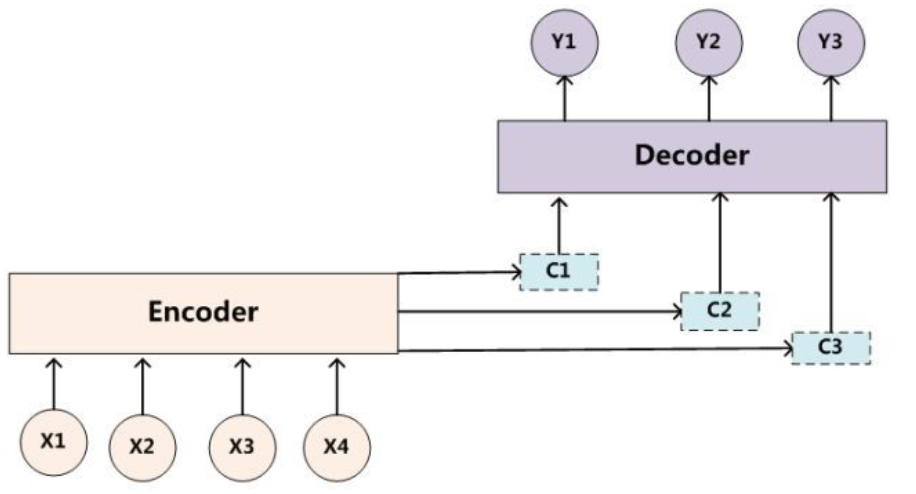C i = ∑ j = 0 T x a i j f ( x j ) C_i=\sum_{j=0}^{T_x}{a_{ij}f(x_j)}

a i j = e x p ( e i j ) ∑ k = 1 T x e x p ( e i k ) a_{ij}=\frac{exp(e_{ij})}{\sum_{k=1}^{T_x}exp(e_{ik})}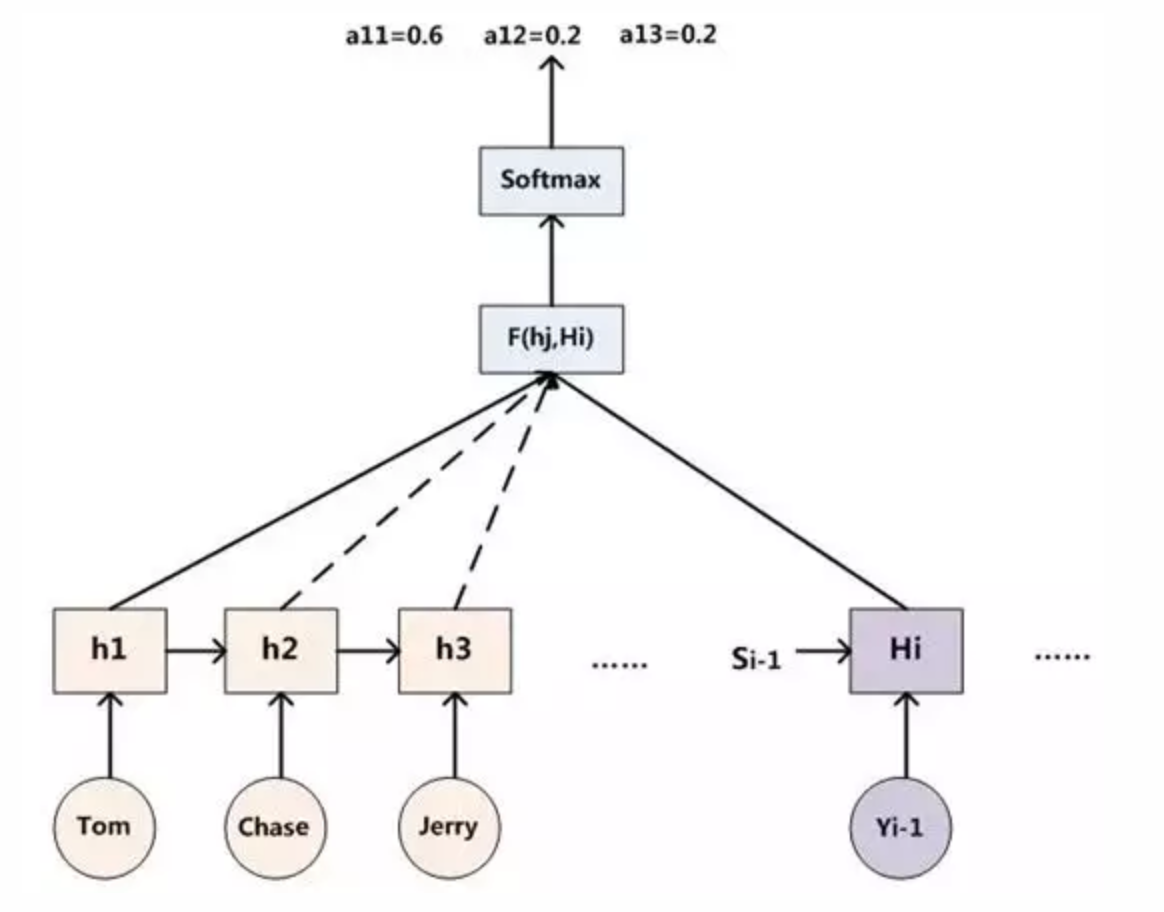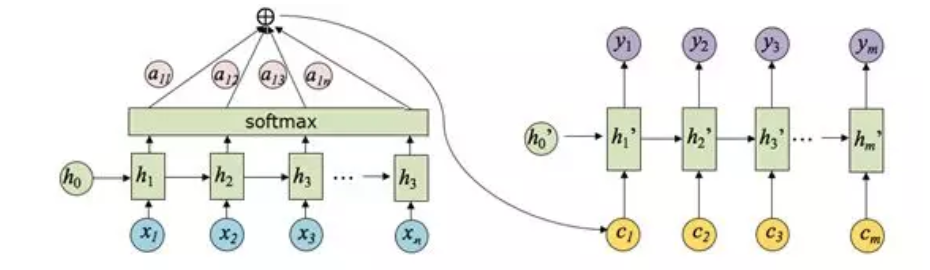① 对 RNN 的输出计算注意程度，通过计算最终时刻的向量与任意 i 时刻向量的权重，通过 softmax 计算出得到注意力偏向分数，如果对某一个序列特别注意，那么计算的偏向分数将会比较大。
② 计算 Encoder 中每个时刻的隐向量
③ 将各个时刻对于最后输出的注意力分数进行加权，计算出每个时刻 i 向量应该赋予多少注意力
④ decoder 每个时刻都会将 ③ 部分的注意力权重输入到 Decoder 中，此时 Decoder 中的输入有：经过注意力加权的隐藏层向量，Encoder 的输出向量，以及 Decoder 上一时刻的隐向量
⑤ Decoder 通过不断迭代，Decoder 可以输出最终翻译的序列。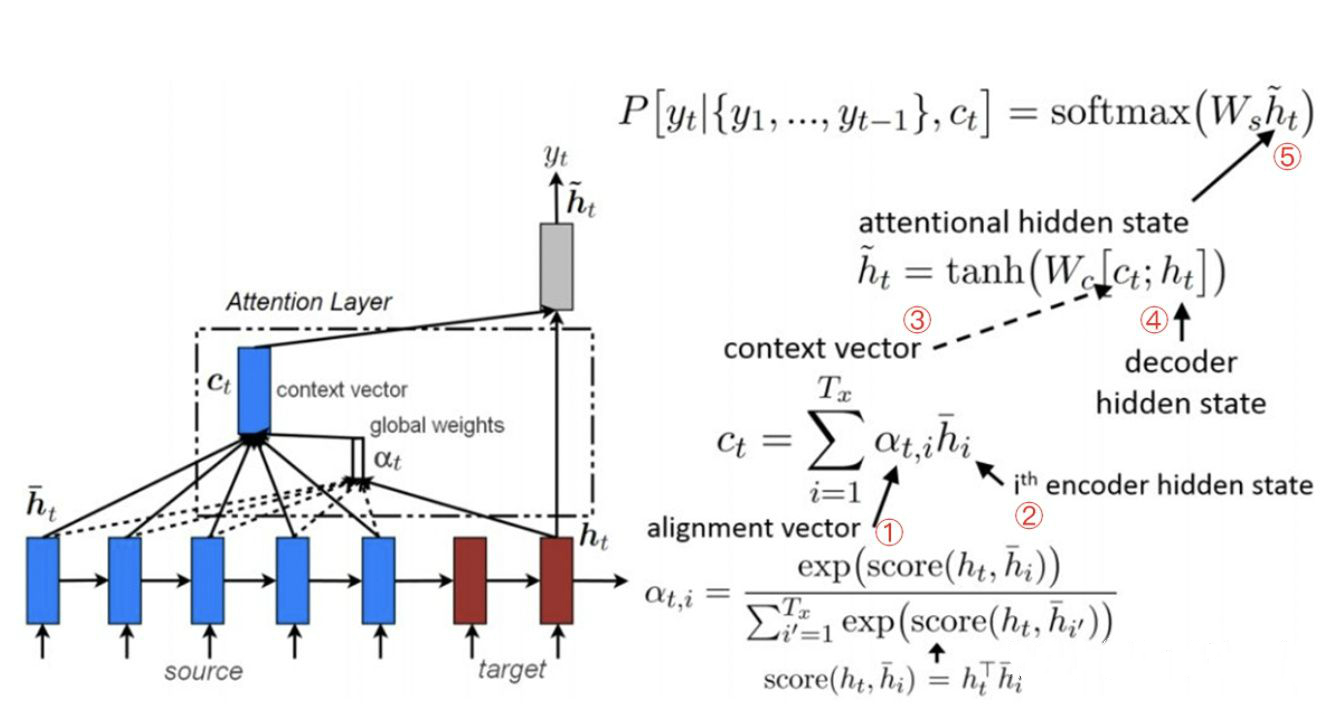# 二、 NMT领域Attention

NMT的先驱是Kalchbrenner and Blunsom (2013)， Sutskever et. al (2014)和Cho. et. al (2014b)，其中比较熟悉的框架是来自Sutskever et. al.的序列到序列(seq2seq)模型。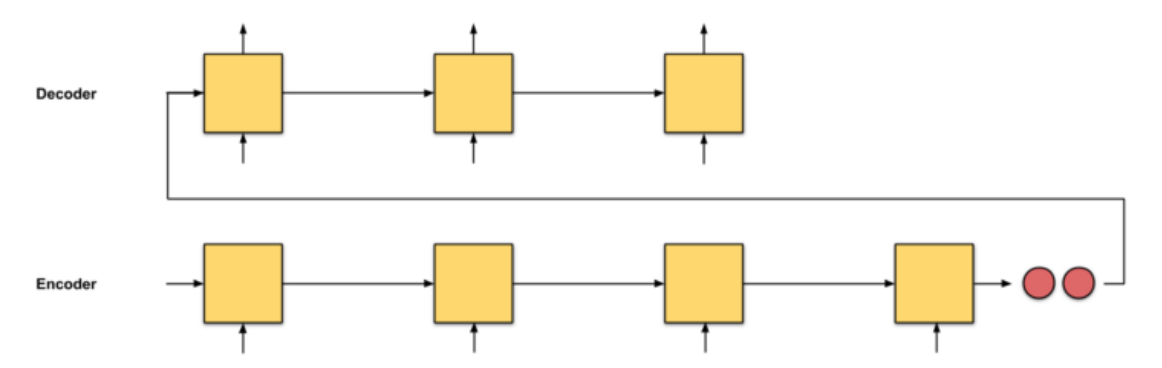seq2seq的问题是，解码器从编码器接收到的唯一信息是编码器的最后隐藏状态（图中的红色向量）这是一个向量表示，类似于输入序列的数值摘要。在长文本中，我们期望解码器只使用这一个向量表示(希望它“充分描述输入序列”)来输出翻译是不现实的。这可能会导致灾难性的遗忘。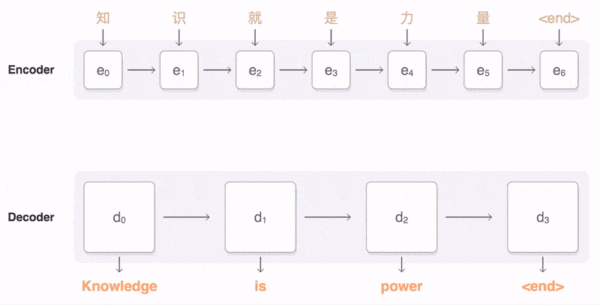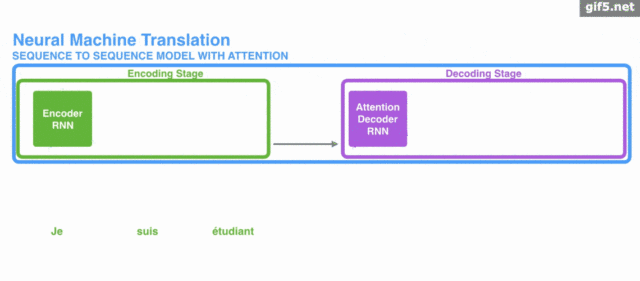# 三、主要代码实现

## 3.1 Encoder

class Encoder(tf.keras.Model):
def __init__(self, hidden_size=1024, max_sequence_len=30, batch_size=batch_size, embedding_dim=256, vocab_size=5000):
super(Encoder, self).__init__()
self.embedding_dim = embedding_dim
self.vocab_size = vocab_size
self.max_sequence_len = max_sequence_len
self.hidden_size = hidden_size
self.batch_size = batch_size

self.embedding_layer = Embedding(
input_dim=self.vocab_size, output_dim=self.embedding_dim)
self.GRU_1 = GRU(units=hidden_size, return_sequences=True)
self.GRU_2 = GRU(units=hidden_size,
return_sequences=True, return_state=True)

def initial_hidden_state(self):
return tf.zeros(shape=(self.batch_size, self.hidden_size))

def call(self, x, initial_state, training=False):
x = self.embedding_layer(x)
x = self.GRU_1(x, initial_state=initial_state)
x, hidden_state = self.GRU_2(x)
return x, hidden_state


## 3.2 Attention

class Attention(tf.keras.Model):
def __init__(self, hidden_size=256):
super(Attention, self).__init__()
self.fc1 = Dense(units=hidden_size)
self.fc2 = Dense(units=hidden_size)
self.fc3 = Dense(units=1)

def call(self, encoder_output, hidden_state, training=False):
'''hidden_state : h(t-1)'''
y_hidden_state = tf.expand_dims(hidden_state, axis=1)
y_hidden_state = self.fc1(y_hidden_state)
y_enc_out = self.fc2(encoder_output)

#get a_ij
y = tf.keras.backend.tanh(y_enc_out + y_hidden_state)
attention_score = self.fc3(y)
attention_weights = tf.keras.backend.softmax(attention_score, axis=1)

#get c_i
context_vector = tf.multiply(encoder_output, attention_weights)
context_vector = tf.reduce_sum(context_vector, axis=1)

return context_vector, attention_weights


## 3.3 Decoder

class Decoder(tf.keras.Model):
def __init__(self, hidden_size=1024, max_sequence_len=30, batch_size=batch_size, embedding_dim=256, vocab_size=5000):
super(Decoder, self).__init__()
self.embedding_dim = embedding_dim
self.vocab_size = vocab_size
self.max_sequence_len = max_sequence_len
self.hidden_size = hidden_size
self.batch_size = batch_size

self.embedding_layer = Embedding(
input_dim=self.vocab_size, output_dim=self.embedding_dim)
self.GRU = GRU(units=hidden_size,
return_sequences=True, return_state=True)
self.attention = Attention(hidden_size=self.hidden_size)
self.fc = Dense(units=self.vocab_size)

def initial_hidden_state(self):
return tf.zeros(shape=(self.batch_size, self.hidden_size))

def call(self, x, encoder_output, hidden_state, training=False):
x = self.embedding_layer(x)
context_vector, attention_weights = self.attention(
encoder_output, hidden_state, training=training)
contect_vector = tf.expand_dims(context_vector, axis=1)
x = tf.concat([x, contect_vector], axis=-1)
x, curr_hidden_state = self.GRU(x)
x = tf.reshape(x, shape=[self.batch_size, -1])
x = self.fc(x)
return x, curr_hidden_state, attention_weights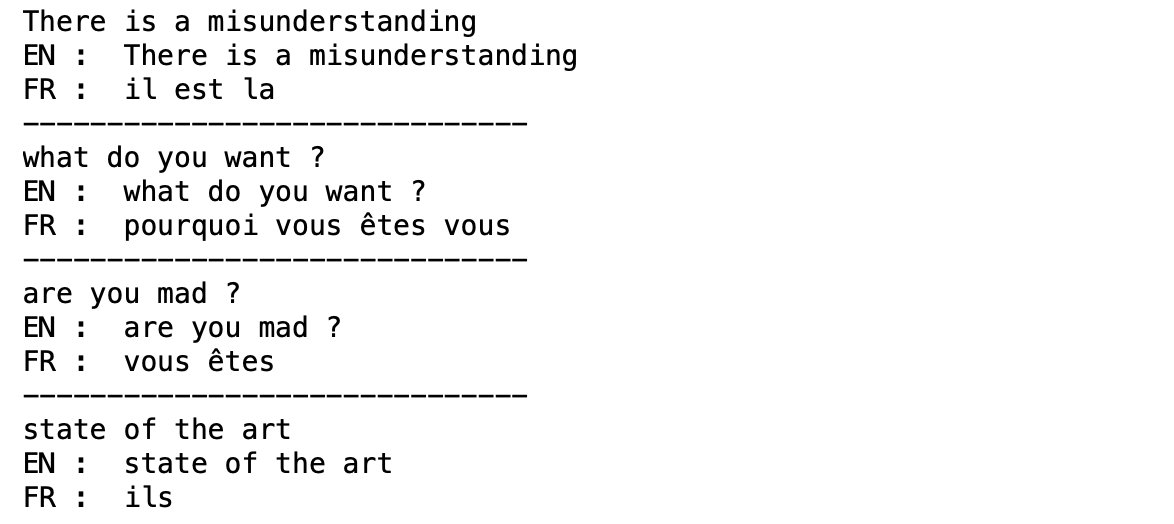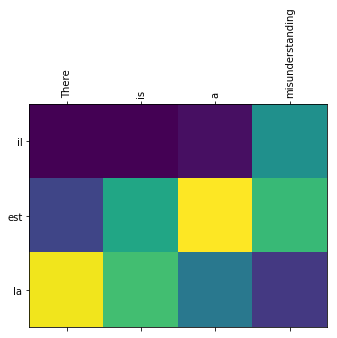https://zhuanlan.zhihu.com/p/31547842

Attention可视化：https://jalammar.github.io/visualizing-neural-machine-translation-mechanics-of-seq2seq-models-with-attention/
Intuitive Understanding of Attention Mechanism in Deep Learning：
https://towardsdatascience.com/intuitive-understanding-of-attention-mechanism-in-deep-learning-6c9482aecf4f
kaggle翻译例子1：https://www.kaggle.com/nikhilxavier/english-to-hindi-machine-translation-attention
kaggle翻译例子2：https://www.kaggle.com/harishreddy18/english-to-french-translation
Go from the basics - Attention mechanism, transformers, BERT：11-122174
06-11619
04-118107
05-24323
06-081172
06-02984